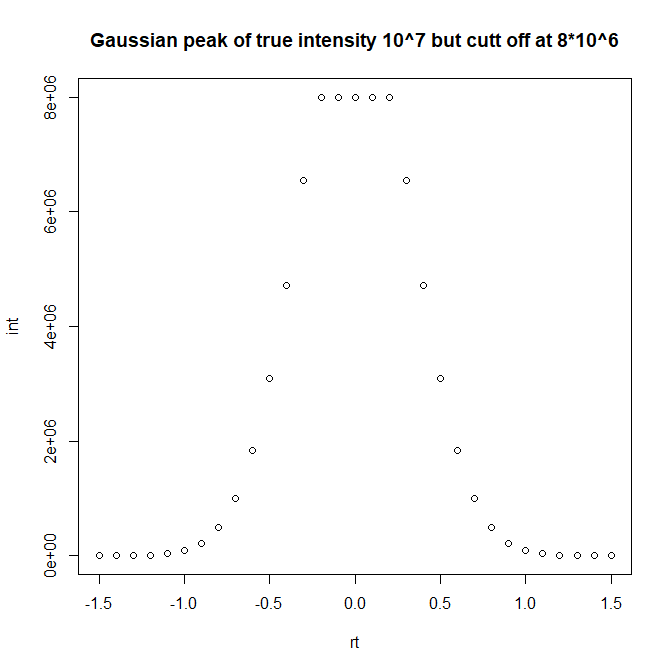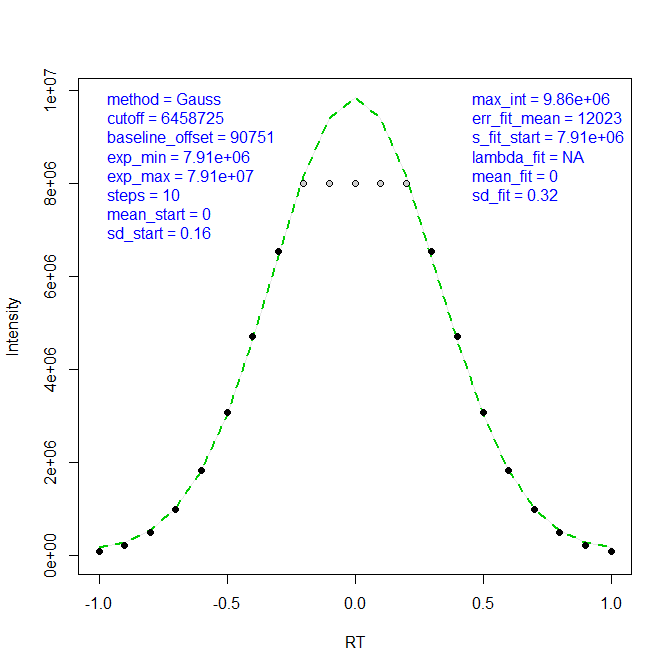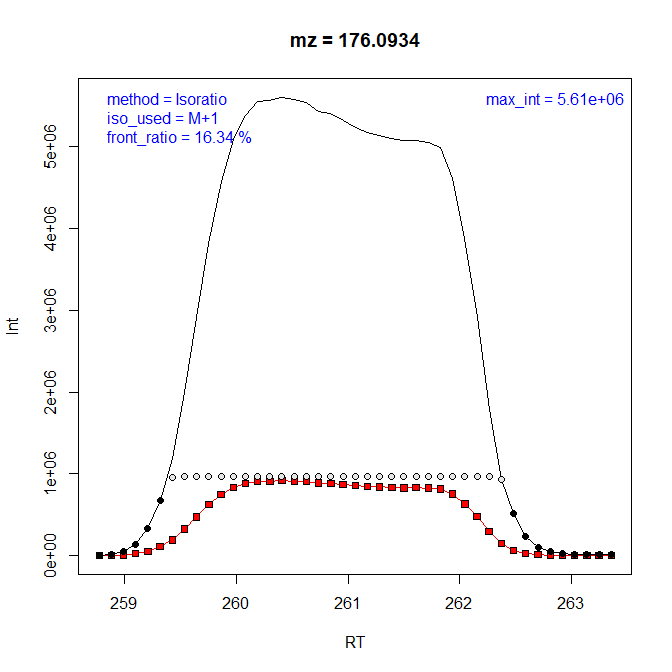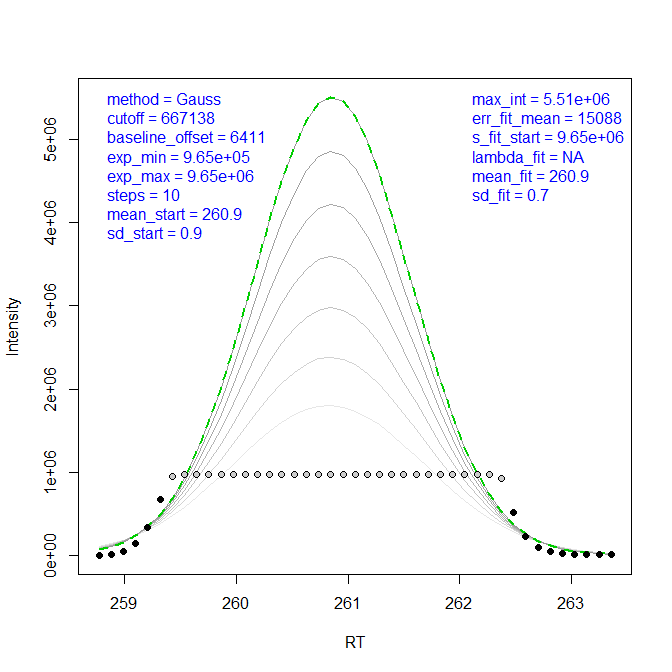CorrectOverloadedPeaks: a computational tool for automatic correction of overloaded signals in GC-APCI-MS

20.08.2016

This short Vignette will show how to correction overloaded signals in (i) an artificial test case and (ii) a provided real data set. To achieve this we need to load the package functions as well as a small data example in xcmsRaw format.

data("mzXML_data")

Let’s model a typical overloaded signal occuring frequently in GC-APCI-MS using the provided function .

pk <- CorrectOverloadedPeaks::ModelGaussPeak(height=10^7, width=3, scan_rate=10, e=0, ds=8*10^6, base_line=10^2)
plot(pk, main="Gaussian peak of true intensity 10^7 but cutt off at 8*10^6")Now we roughly estimate peak boarders before applying the provided function to correct peak data.

idx <- pk[,"int"]>0.005 * max(pk[,"int"])
tmp <- CorrectOverloadedPeaks::FitGaussPeak(x=pk[idx,"rt"], y=pk[idx,"int"], silent=FALSE, xlab="RT", ylab="Intensity")
##  "Number of converging sollutions: 10, keeping 1"The generated QC plot does show the optimal solution found (green line), indicating the substituted intensity values (grey circles) and obtained parameters (blue text) including the probably peak height (max_int=9.7*10^6) being very close to the true peak height (10^7). Now let’s extend this simplified process to peaks from a real data set. The following function call will generate (i) a PDF in the working directory with QC-plots for 10 peaks from 5 chromatographic regions, (ii) processing information output to the console and (iii) a new file “cor_df_all.RData” in the working directory containing all extracted but non-corrected mass traces.

##
## Processing... mzXML_data
##
## Trying to correct 1 overloaded regions.
##  "Processing Region/Mass: 1 / 1"
##  "Number of converging sollutions: 192, keeping 153"
##  "Processing Region/Mass: 1 / 2"
##  "Number of converging sollutions: 194, keeping 36"
##  "Storing non-corrected data information in 'cor_df_all.RData'"

Let’s load these non-corrected mass traces for further visualization of package capabilities. For instance we can reprocess peak 2 from region 4 using the isotopic ratio approach:

##     Scan      RT      mz0   int0      mz1   int1 modified
## 169  169 258.771 176.0924   6411 177.0939   1027    FALSE
## 170  170 258.880 176.0923  17631 177.0941   3353    FALSE
## 171  171 258.989 176.0920  53682 177.0938   8674    FALSE
## 172  172 259.098 176.0919 141884 177.0935  23987    FALSE
## 173  173 259.208 176.0921 335177 177.0937  52495    FALSE
## 174  174 259.316 176.0924 673549 177.0938 111346    FALSE